# Linear prediction

(diff) ← Older revision | Latest revision (diff) | Newer revision → (diff)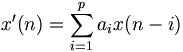$x'(n)=\sum_{i=1}^p a_i x(n-i)$
The trick with linear prediction is to choose the coefficients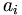$a_i$ in such a way that the difference between the estimated value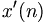$x'(n)$ and the real value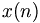$x(n)$ is as small as possible. There are several ways of doing this, but one simple, commonly used (because of its simplicity, not its performance) method is to assume that the signal more or less follows an n-th order polynomial and simply use fixed coefficients (for example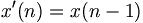$x'(n)=x(n-1)$ or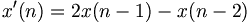$x'(n)=2x(n-1)-x(n-2)$, where the first assumes a function of the form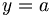$y=a$ and the second assumes a function of the form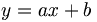$y=ax+b$).